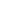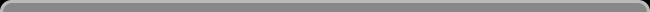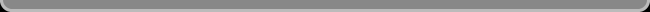Navigation: LaesieWorksImage Compression HomepageThis pageTHE SHORTEST CODE

The shortest code is: no code.
The absence of code can be information also.
For instance, if the rule is "if no code, then make a value that is in between the previous and next value".
140.(nothing).142.145.(nothing).149.
becomes
140.141.142.145.147.149.
But writing no code in a file is kind of impossible.
Missing code could maybe work.

The shortest code that can be written is one 'bit'.
One bit is the smallest piece of information, and it has two states, commonly named "0" and "1". A better way to describe these two states is "no" and "yes".
All longer codes, are combinations of two or more bits.

This is NOT an efficiënt way of noting values:
0 = 0
1 = 1
2 = 11
3 = 111
4 = 1111
5 = 11111
6 = 111111
7 = 1111111
Not efficiënt because a bit has two states, while here is only one stateused (except for zero). The powerful thing of bits is the amount of unique combinations you can make with them.

One bit has only two states or "combinations":
0
1
Two bits have 4 combinations:
00
01
10
11
Three bits have 8 combinations:
000
001
010
011
100
101
110
111

Here a list of the number of bits plus the amount of combinations:
1 = 2
2 = 4
3 = 8
4 = 16
5 = 32
6 = 64
7 = 128
8 = 256
... (this can continue for ever)
Each time one bit is added, the amount of unique combinations doubles!
An easy way to calculate this is: 2 (the 2 states of one bit) to the power of the amount of bits.
2^1 = 2
2^2 = 4
2^3 = 8
2^4 = 16
2^5 = 32
2^6 = 64
2^7 = 128
2^8 = 256

Fixed Length
One normal RGB pixels has a code of 3*8 = 24 bits
2^24 = 16777216 combinations

If you were to write down all the combinations of 24 bits, you'll notice right away that a fixed length for each combination is simple, but not efficiënt:
0 = 000000000000000000000000
1 = 000000000000000000000001
2 = 000000000000000000000010
3 = 000000000000000000000011
4 = 000000000000000000000100
5 = 000000000000000000000101
6 = 000000000000000000000110
Also is there a limit to the amount of unique combinations. 24 bits can't generate more than 16777216 unique combinations.

Variable Length
Using codes of variable length can reduce the amount of bits needed, and the amount of unique combinations it can generate can be unlimited.
 Decimal Fixed length Variable length 0 1 2 3 4 5 6 7 8 9 10 11 12 13 14 15 0000 0001 0010 0011 0100 0101 0110 0111 1000 1001 1010 1011 1100 1101 1110 1111 0 1 00 01 10 11 000 001 010 011 100 101 110 111 0000 0001

The thing with a variable length though, is that you don't know where the code stops and starts. One solution is to use a 'prefix', a short code that tells how long the next code will be. This prefix code can be placed inside the code, or separate in front.
An example of: a 3 bit prefix, the code, and the result:
Prefix code: 2 - 4 - 3 - 5 - 2 - 8 - 4 - 2 (which of course are written in digital code)
Code: 010010110100101111010010000100
Code result: 01 - 0010 - 110 - 10010 - 11 - 11010010 - 0001 - 00

The amount of possible combinations is the depending on the length of the prefix.
If the prefix is 1 bit, there are 2 lengths possible:
prefix - code
0 - 0
0 - 1
1 - 00
1 - 01
1 - 10
1 - 11
A total of 6 combinations, at an average cost of 16/6 = 2.6667 bits per code.
To get 6 combinations with a fixed length, that would need to be 3 bits per code. Though with 3 bits there are 8 possible combinations instead of 6.

This is how to calculate the amount of possible combinations with a certain amount of prefix bits:
1 prefix bit
(2^1) + (2^2) = 6
2 prefix bits
(2^1) + (2^2) + (2^3) + (2^4) = 30
3 prefix bits
(2^1) + (2^2) + (2^3) + (2^4) + (2^5) + (2^6) + (2^7) + (2^8) = 510
And when the need for huge amounts of combinations is there, you can also add a prexfix code to the prefix code!

Prefix-free Codes
An other solution is to use a code that can not confuse.
'Huffman coding' uses codes of variable length that can not confuse, so it can function without a prefix code.

More Short
A common way to get shorter codes, is to assign the shortest codes to the most common colors. Most often relative values (the change of color relative to a previous pixel). It doesn't create shorter codes, but as the longer codes are rarely used, the total amount of bits is reduced.

For this to work, a colors-index must be added to the file. This index contains a list that assigns the shortest codes to the most common colors, and the colors that do not appear in the photo or movie, will not get a code at all.
If all values appear in almost equal amounts and in quite random order, as is the case in data that is already compressed, than it makes no sense to build such an index.
Also does storing the index cost bits. Working with an colors-index must remove more bits than it adds, for it to be efficient.

Dynamic Index
A fixed index has a certain number of specific colors indexed. All the colors that appear in a movie for example, in the order of how common they are.
But most frames of a movie aren't equal to the average of the whole movie, and different areas within one frame aren't equal to the average of their frame. One frame doesn't contain as much colors as the whole movie, and one area within one frame doesn't contain as much colors as its frame. So it makes sense to work with a dynamic index that is always fit for the frame and area where the code is at, to give the most common colors -at that time- the shortest codes.

 Giesbert Nijhuis